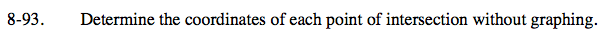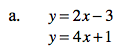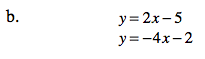Home > ACC7 > Chapter cc38 > Lesson cc38.2.3 > Problem8-93

8-93.
1. Determine the coordinates of each point of intersection without graphing. Homework Help ✎

1. y = 2x − 3

y = 4x + 1

2. y = 2x − 5

y = −4x −2Use the Equal Values Method.

Set both equations equal to each other.
2x − 3 = 4x + 1

Get all the x terms on one side of the equation.
−2x = 4

Divide both sides by −2 to solve for x.

x = −2
Substitute this value into one of the original equations to solve for y.

(−2, −7)Follow the steps in part (a).

$\left(\frac{1}{2}, -4\right)$